# cxhull

2022-10-25

The purpose of the `cxhull` package is to compute the convex hull of a set of points in arbitrary dimension. Its main function is named `cxhull`.

The output of the `cxhull` function is a list with the following fields.

• `vertices`: The vertices of the convex hull. Each vertex is given with its neighbour vertices, its neighbour ridges and its neighbour facets.

• `edges`: The edges of the convex hull, given as pairs of vertices identifiers.

• `ridges`: The ridges of the convex hull, i.e. the elements of the convex hull of dimension `dim-2`. Thus the ridges are just the vertices in dimension 2, and they are the edges in dimension 3.

• `facets`: The facets of the convex hull, i.e. the elements of the convex hull of dimension `dim-1`. Thus the facets are the edges in dimension 2, and they are the faces of the convex polyhedron in dimension 3.

• `volume`: The volume of the convex hull (area in dimension 2, volume in dimension 3, hypervolume in higher dimension).

Let’s look at an example. The points we take are the vertices of a cube and the center of this cube (in the first row):

``````library(cxhull)
points <- rbind(
c(0.5, 0.5, 0.5),
c(0, 0, 0),
c(0, 0, 1),
c(0, 1, 0),
c(0, 1, 1),
c(1, 0, 0),
c(1, 0, 1),
c(1, 1, 0),
c(1, 1, 1)
)
hull <- cxhull(points)``````

Obviously, the convex hull of these points is the cube. We can quickly see that the convex hull has 8 vertices, 12 edges, 12 ridges, 6 facets, and its volume is 1:

``````str(hull, max = 1)
## List of 5
##  \$ vertices:List of 8
##  \$ edges   : int [1:12, 1:2] 2 2 2 3 3 4 4 5 6 6 ...
##  \$ ridges  :List of 12
##  \$ facets  :List of 6
##  \$ volume  : num 1
##  - attr(*, "3d")= logi TRUE``````

Each vertex, each ridge, and each facet has an identifier. A vertex identifier is the index of the row corresponding to this vertex in the set of points passed to the `cxhull` function. It is given in the field `id` of the vertex:

``````hull[["vertices"]][]
## \$id
##  2
##
## \$point
##  0 0 0
##
## \$neighvertices
##  3 4 6
##
## \$neighridges
##  1 2 5
##
## \$neighfacets
##  1 2 4``````

Also, the list of vertices is named with the identifiers:

``````names(hull[["vertices"]])
##  "2" "3" "4" "5" "6" "7" "8" "9"``````

Edges are given as a matrix, each row representing an edge given as a pair of vertices identifiers:

``````hull[["edges"]]
##       [,1] [,2]
##  [1,]    2    3
##  [2,]    2    4
##  [3,]    2    6
##  [4,]    3    5
##  [5,]    3    7
##  [6,]    4    5
##  [7,]    4    8
##  [8,]    5    9
##  [9,]    6    7
## [10,]    6    8
## [11,]    7    9
## [12,]    8    9``````

The ridges are given as a list:

``````hull[["ridges"]][]
## \$id
##  1
##
## \$ridgeOf
##  1 4
##
## \$vertices
##  2 4``````

The `vertices` field provides the vertices identifiers of the ridge. A ridge is between two facets; the identifiers of these facets are given in the field `ridgeOf`.

Facets are given as a list:

``````hull[["facets"]][]
## \$vertices
##  8 4 6 2
##
## \$edges
##      [,1] [,2]
## [1,]    2    4
## [2,]    2    6
## [3,]    4    8
## [4,]    6    8
##
## \$ridges
##  1 2 3 4
##
## \$neighbors
##  2 3 4 5
##
## \$volume
##  1
##
## \$center
##  0.5 0.5 0.0
##
## \$normal
##   0  0 -1
##
## \$offset
##  0
##
## \$family
##  NA
##
## \$orientation
##  1``````

There is no `id` field for the facets: the integer `i` is the identifier of the `i`-th facet of the list.

The `orientation` field has two possible values, `1` or `-1`, it indicates the orientation of the facet. See the plotting example below.

Here, the `family` field is `NA` for every facet:

``````sapply(hull[["facets"]], `[[`, "family")
##  NA NA NA NA NA NA``````

This field has a possibly non-missing value only when one requires the triangulation of the convex hull:

``````thull <- cxhull(points, triangulate = TRUE)
sapply(thull[["facets"]], `[[`, "family")
##    0  0  2  2  4  4  6  6  8  8 10 10``````

The hull is triangulated into 12 triangles: each face of the cube is triangulated into two triangles. Therefore one gets six different families, each one consisting of two triangles: two triangles belong to the same family mean that they are parts of the same facet of the non-triangulated hull.

## Ordering the vertices

Observe the vertices of the first face of the cube:

``````hull[["facets"]][][["vertices"]]
##  8 4 6 2``````

They are given as `8-4-6-2`. They are not ordered, in the sense that `4-6` and `2-8` are not edges of this face:

``````( face_edges <- hull[["facets"]][][["edges"]] )
##      [,1] [,2]
## [1,]    2    4
## [2,]    2    6
## [3,]    4    8
## [4,]    6    8``````

One can order the vertices as follows:

``````polygonize <- function(edges){
nedges <- nrow(edges)
vs <- edges[1, ]
v <- vs
edges <- edges[-1, ]
for(. in 1:(nedges-2)){
j <- which(apply(edges, 1, function(e) v %in% e))
v <- edges[j, ][which(edges[j, ] != v)]
vs <- c(vs, v)
edges <- edges[-j, ]
}
vs
}
polygonize(face_edges)
##  2 4 8 6``````

Instead of using this function, use the `hullMesh` function. It returns the vertices of the convex hull and its faces with ordered indices.

## The `cxhullEdges` function

The `cxhull` function returns a lot of information about the convex hull. If you only want to find the edges of the convex hull, use the `cxhullEdges` function instead, for a speed gain and less memory consumption. For example, the `cxhull` function fails on my laptop for the E8 root polytope, while the `cxhullEdges` function works (but it takes a while).

## Plotting a 3-dimensional hull

The package provides the function `plotConvexHull3d` to plot a triangulated 3-dimensional hull with rgl. Let’s take an icosidodecahedron as example:

``````library(cxhull)
library(rgl)
# icosidodecahedron
phi <- (1+sqrt(5))/2
vs1 <- rbind(
c(0, 0, 2*phi),
c(0, 2*phi, 0),
c(2*phi, 0, 0)
)
vs1 <- rbind(vs1, -vs1)
vs2 <- rbind(
c( 1,  phi,  phi^2),
c( 1,  phi, -phi^2),
c( 1, -phi,  phi^2),
c(-1,  phi,  phi^2),
c( 1, -phi, -phi^2),
c(-1,  phi, -phi^2),
c(-1, -phi,  phi^2),
c(-1, -phi, -phi^2)
)
vs2 <- rbind(vs2, vs2[, c(2, 3, 1)], vs2[, c(3, 1, 2)])
points <- rbind(vs1, vs2)
# computes the triangulated convex hull:
hull <- cxhull(points, triangulate = TRUE)``````
``````# plot:
open3d(windowRect = c(50, 50, 562, 562))
view3d(10, 80, zoom = 0.7)
plotConvexHull3d(
hull, facesColor = "orangered", edgesColor = "yellow",
)``````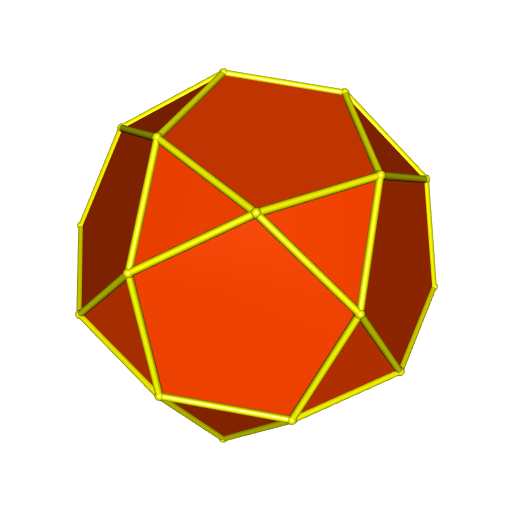## Facets orientation

The `plotConvexHull3d` function calls the `TrianglesXYZ` function, which takes care of the orientation of the facets. Indeed, with the code below, we see the whole convex hull while we hide the back side of the triangles:

``````triangles <- TrianglesXYZ(hull)
open3d(windowRect = c(50, 50, 562, 562))
view3d(10, 80, zoom = 0.7)
triangles3d(triangles, color = "green", back = "culled")``````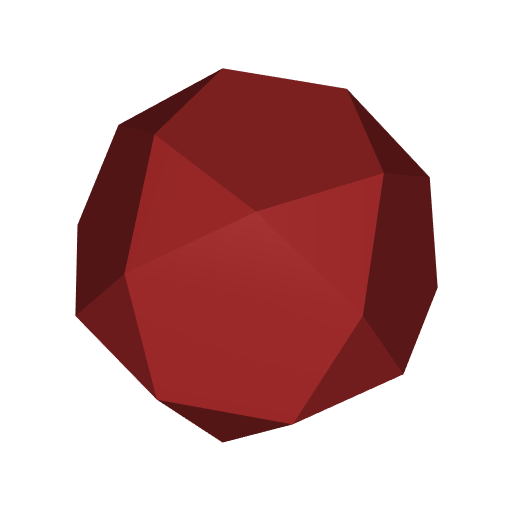The `orientation` field of a facet indicates its orientation (`1` or `-1`).

## Plotting with multiple colors

There are three possiblities for the `facesColor` argument of the `plotConvexHull3d` function. We have already seen the first one: a single color. The second possibiity is to assign a color to each triangle of the hull. There are 56 triangles:

``````length(hull[["facets"]])
##  56``````

So we specify 56 colors:

``````library(randomcoloR)
colors <- distinctColorPalette(56)
open3d(windowRect = c(50, 50, 562, 562))
view3d(10, 80, zoom = 0.7)
plotConvexHull3d(
hull, facesColor = colors, edgesColor = "yellow",
)``````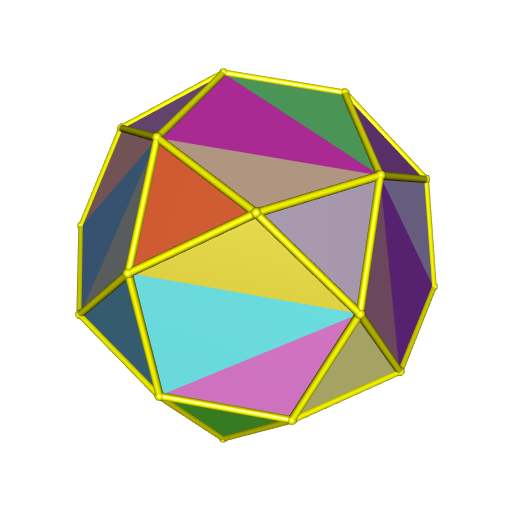The third possibility is to assign a color to each face of the convex hull. There are 32 faces:

``````summary <- hullSummary(hull)
attr(summary, "facets")
##  "20 triangular facets, 12 other facets"``````
``````library(randomcoloR)
colors <- distinctColorPalette(32)
open3d(windowRect = c(50, 50, 562, 562))
view3d(10, 80, zoom = 0.7)
plotConvexHull3d(
hull, facesColor = colors, edgesColor = "yellow",
)``````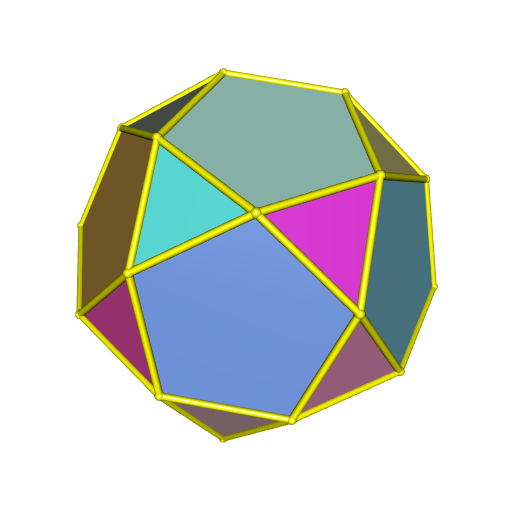Finally, instead of using the `facesColor` argument, you can use the `palette` argument, which allows to decorate the faces with a color gradient.

``````open3d(windowRect = c(50, 50, 562, 562))
view3d(10, 80, zoom = 0.7)
plotConvexHull3d(
hull, palette = hcl.colors(256, "BuPu"), bias = 0.25,
)``````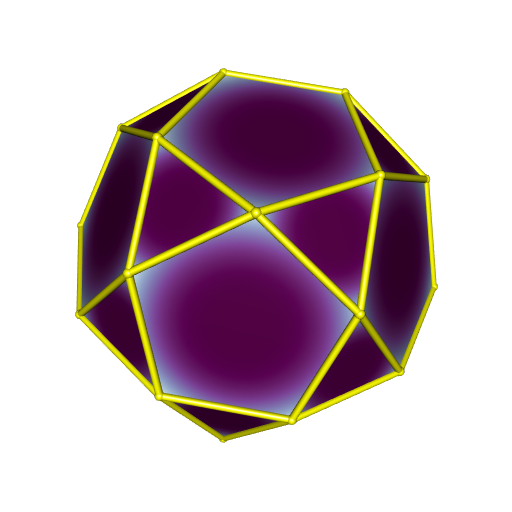# A four-dimensional example

Now, to illustrate the `cxhull` package, we deal with a four-dimensional polytope: the truncated tesseract.

It is a convex polytope whose vertices are given by all permutations of (±1, ±(√2+1), ±(√2+1), ±(√2+1)).

Let’s enter these 64 vertices in a matrix `points`:

``````sqr2p1 <- sqrt(2) + 1
points <- rbind(
c(-1, -sqr2p1, -sqr2p1, -sqr2p1),
c(-1, -sqr2p1, -sqr2p1, sqr2p1),
c(-1, -sqr2p1, sqr2p1, -sqr2p1),
c(-1, -sqr2p1, sqr2p1, sqr2p1),
c(-1, sqr2p1, -sqr2p1, -sqr2p1),
c(-1, sqr2p1, -sqr2p1, sqr2p1),
c(-1, sqr2p1, sqr2p1, -sqr2p1),
c(-1, sqr2p1, sqr2p1, sqr2p1),
c(1, -sqr2p1, -sqr2p1, -sqr2p1),
c(1, -sqr2p1, -sqr2p1, sqr2p1),
c(1, -sqr2p1, sqr2p1, -sqr2p1),
c(1, -sqr2p1, sqr2p1, sqr2p1),
c(1, sqr2p1, -sqr2p1, -sqr2p1),
c(1, sqr2p1, -sqr2p1, sqr2p1),
c(1, sqr2p1, sqr2p1, -sqr2p1),
c(1, sqr2p1, sqr2p1, sqr2p1),
c(-sqr2p1, -1, -sqr2p1, -sqr2p1),
c(-sqr2p1, -1, -sqr2p1, sqr2p1),
c(-sqr2p1, -1, sqr2p1, -sqr2p1),
c(-sqr2p1, -1, sqr2p1, sqr2p1),
c(-sqr2p1, 1, -sqr2p1, -sqr2p1),
c(-sqr2p1, 1, -sqr2p1, sqr2p1),
c(-sqr2p1, 1, sqr2p1, -sqr2p1),
c(-sqr2p1, 1, sqr2p1, sqr2p1),
c(sqr2p1, -1, -sqr2p1, -sqr2p1),
c(sqr2p1, -1, -sqr2p1, sqr2p1),
c(sqr2p1, -1, sqr2p1, -sqr2p1),
c(sqr2p1, -1, sqr2p1, sqr2p1),
c(sqr2p1, 1, -sqr2p1, -sqr2p1),
c(sqr2p1, 1, -sqr2p1, sqr2p1),
c(sqr2p1, 1, sqr2p1, -sqr2p1),
c(sqr2p1, 1, sqr2p1, sqr2p1),
c(-sqr2p1, -sqr2p1, -1, -sqr2p1),
c(-sqr2p1, -sqr2p1, -1, sqr2p1),
c(-sqr2p1, -sqr2p1, 1, -sqr2p1),
c(-sqr2p1, -sqr2p1, 1, sqr2p1),
c(-sqr2p1, sqr2p1, -1, -sqr2p1),
c(-sqr2p1, sqr2p1, -1, sqr2p1),
c(-sqr2p1, sqr2p1, 1, -sqr2p1),
c(-sqr2p1, sqr2p1, 1, sqr2p1),
c(sqr2p1, -sqr2p1, -1, -sqr2p1),
c(sqr2p1, -sqr2p1, -1, sqr2p1),
c(sqr2p1, -sqr2p1, 1, -sqr2p1),
c(sqr2p1, -sqr2p1, 1, sqr2p1),
c(sqr2p1, sqr2p1, -1, -sqr2p1),
c(sqr2p1, sqr2p1, -1, sqr2p1),
c(sqr2p1, sqr2p1, 1, -sqr2p1),
c(sqr2p1, sqr2p1, 1, sqr2p1),
c(-sqr2p1, -sqr2p1, -sqr2p1, -1),
c(-sqr2p1, -sqr2p1, -sqr2p1, 1),
c(-sqr2p1, -sqr2p1, sqr2p1, -1),
c(-sqr2p1, -sqr2p1, sqr2p1, 1),
c(-sqr2p1, sqr2p1, -sqr2p1, -1),
c(-sqr2p1, sqr2p1, -sqr2p1, 1),
c(-sqr2p1, sqr2p1, sqr2p1, -1),
c(-sqr2p1, sqr2p1, sqr2p1, 1),
c(sqr2p1, -sqr2p1, -sqr2p1, -1),
c(sqr2p1, -sqr2p1, -sqr2p1, 1),
c(sqr2p1, -sqr2p1, sqr2p1, -1),
c(sqr2p1, -sqr2p1, sqr2p1, 1),
c(sqr2p1, sqr2p1, -sqr2p1, -1),
c(sqr2p1, sqr2p1, -sqr2p1, 1),
c(sqr2p1, sqr2p1, sqr2p1, -1),
c(sqr2p1, sqr2p1, sqr2p1, 1)
)``````

As said before, the truncated tesseract is convex, therefore its convex hull is itself. Let’s run the `cxhull` function on its vertices:

``````library(cxhull)
hull <- cxhull(points)
str(hull, max = 1)
## List of 5
##  \$ vertices:List of 64
##  \$ edges   : int [1:128, 1:2] 1 1 1 1 2 2 2 2 3 3 ...
##  \$ ridges  :List of 88
##  \$ facets  :List of 24
##  \$ volume  : num 541``````

We can observe that `cxhull` has not changed the order of the points:

``````all(names(hull[["vertices"]]) == 1:64)
##  TRUE``````

Let’s look at the cells of the truncated tesseract:

``````table(sapply(hull[["facets"]], function(cell) length(cell[["ridges"]])))
##
##  4 14
## 16  8``````

We see that 16 cells are made of 4 ridges; these cells are tetrahedra. We will draw them later, after projecting the truncated tesseract in the 3D-space.

For now, let’s draw the projected vertices and the edges.

The vertices in the 4D-space lie on the centered sphere with radius √(1+3(√2+1)2).

Therefore, a stereographic projection is appropriate to project the truncated tesseract in the 3D-space.

``````sproj <- function(p, r){
c(p, p, p)/(r - p)
}
ppoints <- t(apply(points, 1,
function(point) sproj(point, sqrt(1+3*sqr2p1^2))))``````

Now we are ready to draw the projected vertices and the edges.

``````edges <- hull[["edges"]]
library(rgl)
open3d(windowRect = c(100, 100, 600, 600))
view3d(45, 45)
spheres3d(ppoints, radius = 0.07, color = "orange")
for(i in 1:nrow(edges)){
shade3d(cylinder3d(rbind(ppoints[edges[i, 1], ], ppoints[edges[i, 2], ]),
radius = 0.05, sides = 30), col = "gold")
}``````Pretty nice.

Now let’s show the 16 tetrahedra. Their faces correspond to triangular ridges. So we get the 64 triangles as follows:

``````ridgeSizes <-
sapply(hull[["ridges"]], function(ridge) length(ridge[["vertices"]]))
triangles <- t(sapply(hull[["ridges"]][which(ridgeSizes == 3)],
function(ridge) ridge[["vertices"]]))
##      [,1] [,2] [,3]
## [1,]    1   17   33
## [2,]    1   17   49
## [3,]    1   33   49
## [4,]   17   33   49
## [5,]   12   44   60
## [6,]   12   28   44``````

``````for(i in 1:nrow(triangles)){
triangles3d(rbind(
ppoints[triangles[i, 1], ],
ppoints[triangles[i, 2], ],
ppoints[triangles[i, 3], ]),
color = "red", alpha = 0.4)
}``````We could also use different colors for the tetrahedra:

``````open3d(windowRect = c(100, 100, 600, 600))
view3d(45, 45)
spheres3d(ppoints, radius= 0.07, color = "orange")
for(i in 1:nrow(edges)){
shade3d(cylinder3d(rbind(ppoints[edges[i, 1], ], ppoints[edges[i, 2], ]),
radius = 0.05, sides = 30), col = "gold")
}
cellSizes <- sapply(hull[["facets"]], function(cell) length(cell[["ridges"]]))
tetrahedra <- hull[["facets"]][which(cellSizes == 4)]
colors <- rainbow(16)
for(i in seq_along(tetrahedra)){
triangles <- tetrahedra[[i]][["ridges"]]
for(j in 1:4){
triangle <- hull[["ridges"]][[triangles[j]]][["vertices"]]
triangles3d(rbind(
ppoints[triangle, ],
ppoints[triangle, ],
ppoints[triangle, ]),
color = colors[i], alpha = 0.4)
}
}``````